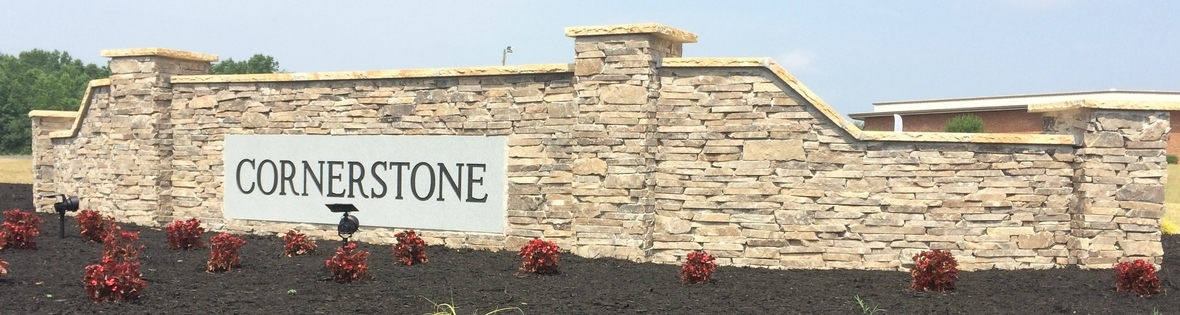Like   Tweet   Pin   +1   in

# JULY 15, 2020

## Cornerstone Conference is a body of IPHC Churches existing to equip ministers and churches to fulfill the Great Commission!

 table div table+table+table+table div table{width:100%;padding:0}table div table+table+table+table div table img{width:96.23%;padding:0;float:none}table div table+table+table+table div table td{width:100%;padding:0 1.88% 18px}/* styles */## By: Joannie DeBrito, Director of Parenting and Youth at Focus on the Family

For those of you with children with special needs, here are some tips that may help your children hold on to the recent gains they’ve made, during the summer or while they’re learning at home.

Supporting Parents of Special Needs Children
For friends and family members, remember that being the parent of a child with special needs takes a lot of energy beyond that which is required of any parent. So, ask the parent of a child with special needs how you can support her (or him) and be ready to pitch in where needed.

Online Therapy Sessions
You might also want to ask if you can arrange therapy sessions via an online video program. If that’s possible, ask the therapist what materials you need to have on hand for the therapy session, before the session starts.

Set A Routine
Remember that most children, including those with special needs, respond well to routines. Typically, repetition yields some good results. So, as much as possible, try to match the routines they’ve gotten used to at school.

Plan for Transitions
Transitions are hard for some children with special needs so, if that’s the case with your child, remember to give him or her notice before you’re going to switch to a new activity. You might want to use a timer so your child can see that the time is winding down for one activity and you’ll be switching soon.

 table div table+table+table+table+table+table+table+table div table{width:100%;padding:0}table div table+table+table+table+table+table+table+table div table img{width:96.23%;padding:0;float:none}table div table+table+table+table+table+table+table+table div table td{width:100%;padding:0 1.88% 18px}/* styles */## Are you a high school or college student that has felt the Lord leading you towards ministry?

Our purpose is to connect you with other students and leaders across the Cornerstone Conference through intentional leadership teaching, and zoom breakouts. Our hope is that you will leave with a deeper understanding of God’s presence, calling and direction for your life.

 /* styles */ The calling of God isn’t always clear or evident, but in our faithfulness to say yes to God He is faithful to position us where we need to be. What if this was an opportunity to find your next step in following God’s calling on your life? We are excited about that opportunity and the privilege we get in partnering together. Whether you are a Pastor, Youth Leader, or Student we would be honored to have you join us for this special weekend. Please take a moment to register for this exciting online event and save your seat! Space is limited for the breakout rooms so please hurry!
 table div table+table+table+table+table+table+table+table+table+table+table+table+table+table div table{width:100%;padding:0}table div table+table+table+table+table+table+table+table+table+table+table+table+table+table div table img{width:96.23%;padding:0;float:none}table div table+table+table+table+table+table+table+table+table+table+table+table+table+table div table td{width:100%;padding:0 1.88% 18px}/* styles */table.module-14{width:78.11%;padding:0}table div table+table+table+table+table+table+table+table+table+table+table+table+table+table+table div table{width:78.11%;float:none;margin-left:auto;margin-right:auto;padding:0}table div table+table+table+table+table+table+table+table+table+table+table+table+table+table+table div table a{border:0 none;text-decoration:none}table div table+table+table+table+table+table+table+table+table+table+table+table+table+table+table div table img{width:100%!important;border:0 none;text-decoration:none}table div table+table+table+table+table+table+table+table+table+table+table+table+table+table+table div table td{width:100%;padding:0}/* styles */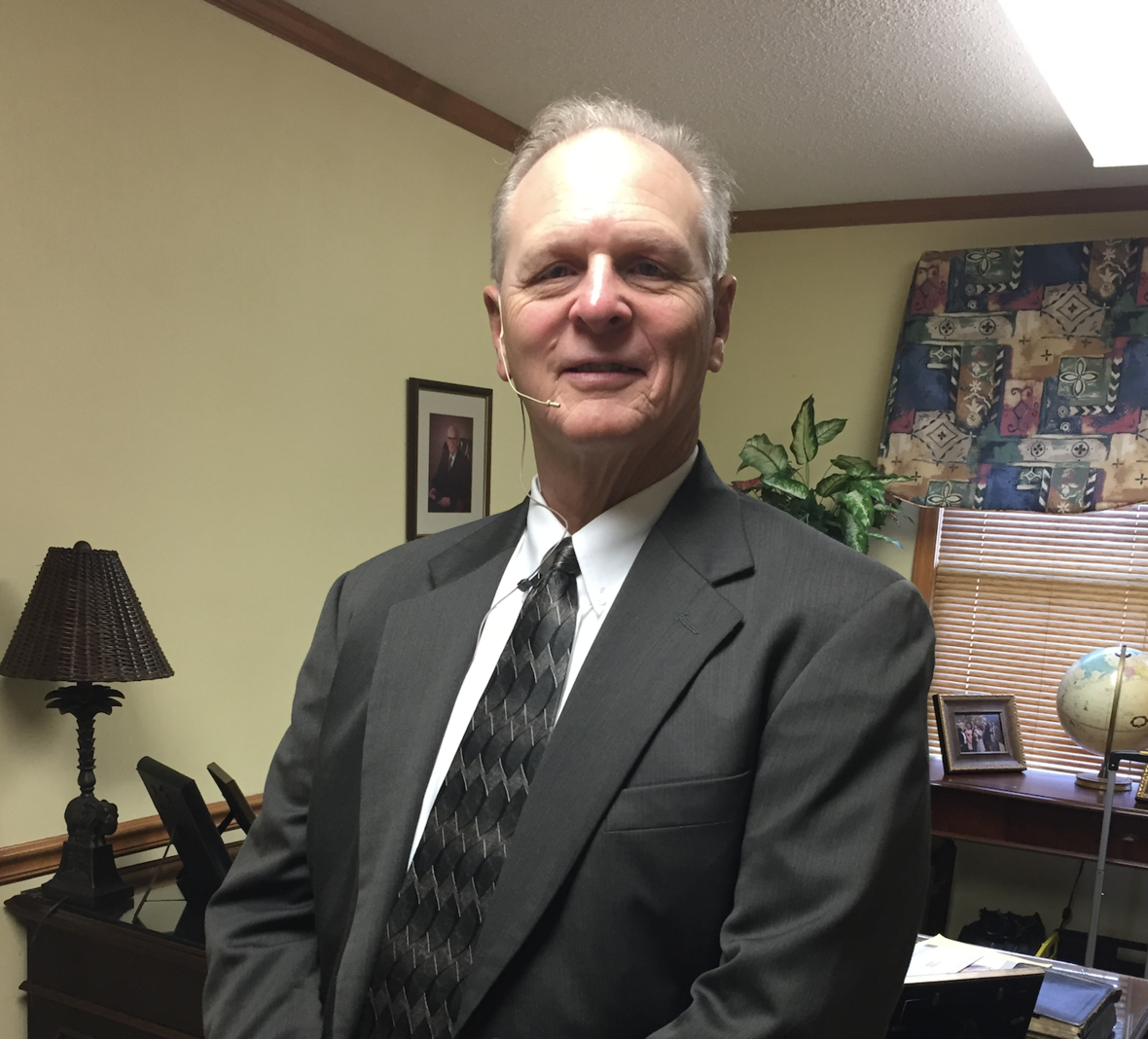# Upcoming Book: I Surrender

## By: Mike Springston

Have you ever looked at the events of your life and wondered, “Why is this happening to me?” We all do but rarely do we stop to consider where these events are trying to lead us.

Mike Springston has been a well-recognized innovator in the world of football since his 1990 team exploded on the scene with an amazing new and high-flying offensive system. Again in 2006, Mike invented the Run Pass Option offense that has taken the football worldwide by storm. With all these innovations in football, Mike knew that there was a war going on in his spirit as winning and success never seemed to be enough.

When Mike began to evaluate himself, he realized that God had a better plan for his life if he would only surrender to the plan that God had for him. Mike currently is assigned by the International Pentecostal Holiness Church as the Lead Pastor of a church in Mount Airy, North Carolina.

His book covers questions and topics such as:

• Are you searching for the meaning and purpose of your life?
• Are you facing a major health issue?
• Have you lost a loved one?
• Are you wondering about the relevance of God?
• Are you wondering if there is a plan for your life and for your future?
• Are you trying to make sense out of the events that have happened in your life?
• Are you trying to locate your calling and settle upon a course for your life that will bring contentment?

If you purchase this book at retail value it will be \$21.99 plus shipping and handling. Below are a few special purchasing options Mike is offering:

1. The cost of a single copy will be \$25.00 which includes tax, shipping and handling
2. If you purchase 5 books at \$25.00 which includes tax, shipping and handling and I will give you a 6th book for ½ price with the addition of tax, shipping and handling.
3. If you buy 15 books at \$25.00 which includes tax, shipping and handling I will give you 1 free.
4. For every increment of 15 books the one free offer stands. (ex.75 books purchased would get you 5 free) Of course, shipping and handling will be added to all orders of any size.
 1 The cost of a single copy will be \$25.00 which includes tax, shipping and handling
 2 If you purchase 5 books at \$25.00 which includes tax, shipping and handling and I will give you a 6th book for ½ price with the addition of tax, shipping and handling.
 3 If you buy 15 books at \$25.00 which includes tax, shipping and handling I will give you 1 free.
 4 For every increment of 15 books the one free offer stands. (ex.75 books purchased would get you 5 free) Of course, shipping and handling will be added to all orders of any size.

Mike is currently in the process of placing his personal order with his publisher and desires to include your order in his request.

## Two ways to order:

1. Visit his ministry website at Mikespringstonministries.com and use the PayPal link to complete your order.
2. Email Mike the total number of books you would like to order at Springston56@gmail.com. If you desire to pay by check or money order, please notify him through email, and he will provide you the address to mail your payment.
 1 Visit his ministry website at Mikespringstonministries.com and use the PayPal link to complete your order.
 2 Email Mike the total number of books you would like to order at Springston56@gmail.com. If you desire to pay by check or money order, please notify him through email, and he will provide you the address to mail your payment.
 table div table+table+table+table+table+table+table+table+table+table+table+table+table+table+table+table+table div table{width:100%;padding:0}table div table+table+table+table+table+table+table+table+table+table+table+table+table+table+table+table+table div table img{width:96.23%;padding:0;float:none}table div table+table+table+table+table+table+table+table+table+table+table+table+table+table+table+table+table div table td{width:100%;padding:0 1.88% 18px}/* styles */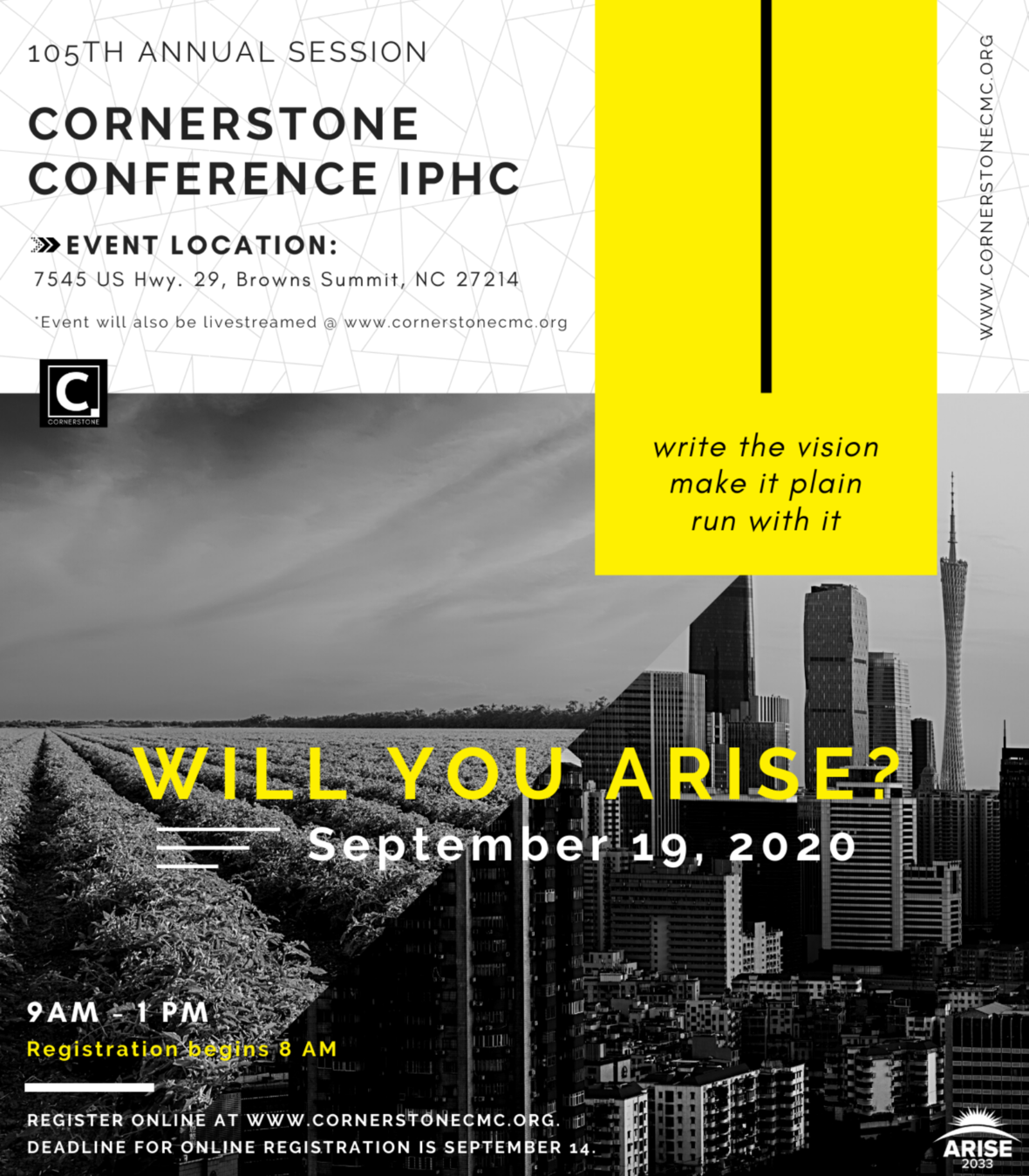## Conference Session begins at 9:00 AM EST (registration will begin at 8 AM)

Please make plans to join us at our Cornerstone Conference Ministry Center for this annual gathering, as we…

▪ Share Vision For Great Commission Ministry
▪ Celebrate Our Arise 2033 New Congregation Goals
▪ Commit To Our IPHC Core Value Of Generosity
▪ License And Ordain New Ministers
▪ Worship And Celebrate Holy Communion Together
 ▪ Share Vision For Great Commission Ministry
 ▪ Celebrate Our Arise 2033 New Congregation Goals
 ▪ Commit To Our IPHC Core Value Of Generosity
 ▪ License And Ordain New Ministers
 ▪ Worship And Celebrate Holy Communion Together

In addition to the State of the Conference Report, Ministerial Credentialing Report and Memoirs Report, our Electoral Procedures Committee will present their report to the Conference in session for approval.

Please note: All ministers are required to participate in this event of the Conference. Spouses are welcome and encouraged to attend.

 table div table+table+table+table+table+table+table+table+table+table+table+table+table+table+table+table+table+table+table+table+table+table div table{width:100%;padding:0}table div table+table+table+table+table+table+table+table+table+table+table+table+table+table+table+table+table+table+table+table+table+table div table img{width:96.23%;padding:0;float:none}table div table+table+table+table+table+table+table+table+table+table+table+table+table+table+table+table+table+table+table+table+table+table div table td{width:100%;padding:0 1.88% 18px}/* styles */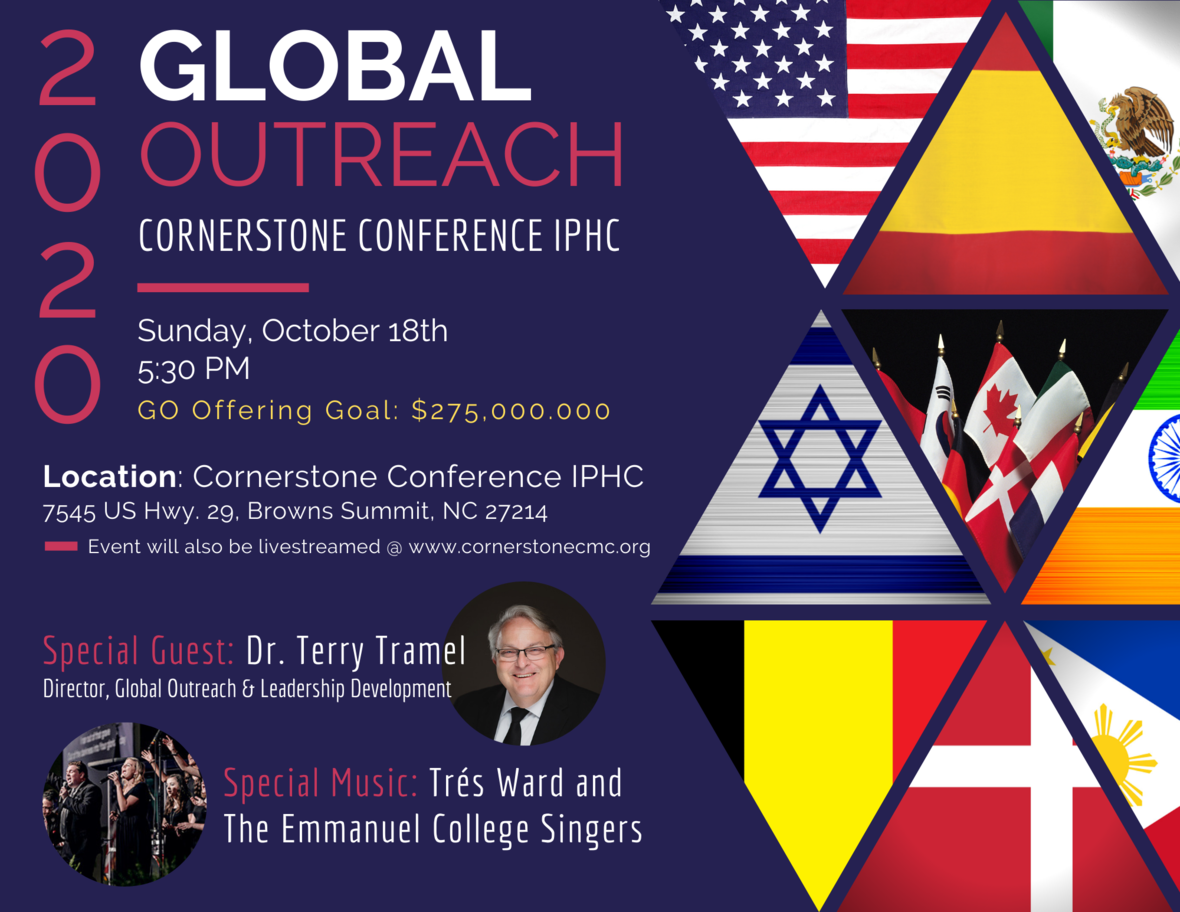## Sunday, October 18th @ 5:30 PM

Our 2020 Global Outreach Goal is \$275,000.000!
Please be in prayer about what the Lord would have you give!

 table div table+table+table+table+table+table+table+table+table+table+table+table+table+table+table+table+table+table+table+table+table+table+table+table+table+table div table{width:100%;padding:0}table div table+table+table+table+table+table+table+table+table+table+table+table+table+table+table+table+table+table+table+table+table+table+table+table+table+table div table img{width:96.23%;padding:0;float:none}table div table+table+table+table+table+table+table+table+table+table+table+table+table+table+table+table+table+table+table+table+table+table+table+table+table+table div table td{width:100%;padding:0 1.88% 18px}/* styles */# Do Not Fear...

## Pam Rooker, Girls' Ministries Director

But now, this is what the LORD says - He who created you, Jacob, He who formed you, Israel: "Do not fear, for I have redeemed you; I have summoned you by name; you are mine. When you pass through the waters, I will be with you; and when you pass through the rivers, they will not sweep over you. When you walk through the fire, you will not be burned; the flames will not set you ablaze.
Isaiah 43:1-2 NIV

 /* styles */ In Isaiah 43:1-2 we are told not to fear. I don’t know about you, but there have been times in my life this year when I have had to remind myself that the Bible tells me do not fear. We must remind ourselves to stand on the promises in God’s word. Promises like Isaiah 43:2 when you pass through the waters, I will be with you; and when you pass through the rivers, they will not sweep over you. When you walk through the fire, you will not be burned; the flames will not set you ablaze. The next time you are feeling overwhelmed by the stress of life, stop and spend some time in God’s word; there you find will peace and assurance.
 table div table+table+table+table+table+table+table+table+table+table+table+table+table+table+table+table+table+table+table+table+table+table+table+table+table+table+table+table+table+table div table{width:100%;padding:0}table div table+table+table+table+table+table+table+table+table+table+table+table+table+table+table+table+table+table+table+table+table+table+table+table+table+table+table+table+table+table div table img{width:96.23%;padding:0;float:none}table div table+table+table+table+table+table+table+table+table+table+table+table+table+table+table+table+table+table+table+table+table+table+table+table+table+table+table+table+table+table div table td{width:100%;padding:0 1.88% 18px}/* styles */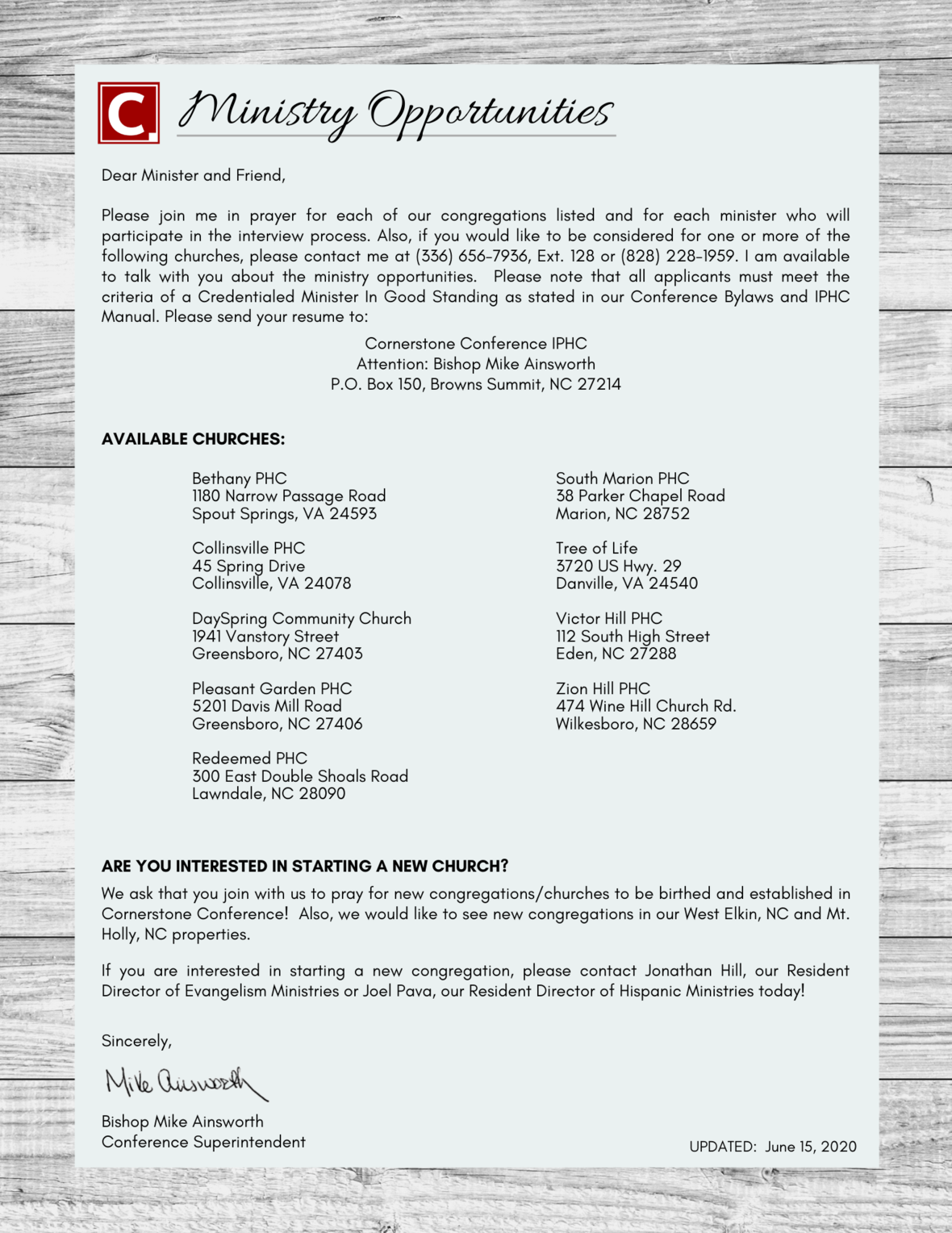## Current Cornerstone Ministry Opportunities

 table div table+table+table+table+table+table+table+table+table+table+table+table+table+table+table+table+table+table+table+table+table+table+table+table+table+table+table+table+table+table+table+table+table div table{width:100%;padding:0}table div table+table+table+table+table+table+table+table+table+table+table+table+table+table+table+table+table+table+table+table+table+table+table+table+table+table+table+table+table+table+table+table+table div table img{width:96.23%;padding:0;float:none}table div table+table+table+table+table+table+table+table+table+table+table+table+table+table+table+table+table+table+table+table+table+table+table+table+table+table+table+table+table+table+table+table+table div table td{width:100%;padding:0 1.88% 18px}/* styles */# Still need your minister ID Card?

## If you have not yet received your Minister ID card please note that we must have a front facing picture of you on file to do so.

Please email Tennille Nichols a jpeg or png file to tnichols@ccrdc.org or mail a photo to her at the Conference Office: PO Box 150 Browns Summit, NC 27214 as soon as possible. If you have any questions please call her at 336-656-7936 ext 110.

 table div table+table+table+table+table+table+table+table+table+table+table+table+table+table+table+table+table+table+table+table+table+table+table+table+table+table+table+table+table+table+table+table+table+table+table+table div table{width:100%;padding:0}table div table+table+table+table+table+table+table+table+table+table+table+table+table+table+table+table+table+table+table+table+table+table+table+table+table+table+table+table+table+table+table+table+table+table+table+table div table img{width:96.23%;padding:0;float:none}table div table+table+table+table+table+table+table+table+table+table+table+table+table+table+table+table+table+table+table+table+table+table+table+table+table+table+table+table+table+table+table+table+table+table+table+table div table td{width:100%;padding:0 1.88% 18px}/* styles */# Latest COVID-19 Resources

Tips for Reopening the Church

Short-Term and Long-Term Effects of COVID-19 on the Church in America
As we slowly emerge out from sheltering in place to a “new” normal, what will the new normal look like for churches in America?
Article by Josh Laxton

Relaunching the Church (Facebook Live Video with Ed Stetzer)

What does it look like to reopen the church?
PowerPoint | Spanish Edition

Podcast: Pastor Resilience (Episode 28)
with Mindy Caliguire, Jimmy Dodd, & Lance Witt

In these seasons of crisis, in our all-important busyness, there is often a subtle temptation to overlook the precious little lives that dwell in our very homes.
Article by Jeff Christopherson and Matt Rogers

Podcast: Children's Ministry in Time of Crisis (Episode 29)
with Mimi Larson, PhD., & Jana Magruder

Podcast: Church Finances in Uncertain Times (Episode 30)
with Todd McMichen

 table div table+table+table+table+table+table+table+table+table+table+table+table+table+table+table+table+table+table+table+table+table+table+table+table+table+table+table+table+table+table+table+table+table+table+table+table+table+table div table{width:100%;padding:0}table div table+table+table+table+table+table+table+table+table+table+table+table+table+table+table+table+table+table+table+table+table+table+table+table+table+table+table+table+table+table+table+table+table+table+table+table+table+table div table img{width:96.23%;padding:0;float:none}table div table+table+table+table+table+table+table+table+table+table+table+table+table+table+table+table+table+table+table+table+table+table+table+table+table+table+table+table+table+table+table+table+table+table+table+table+table+table div table td{width:100%;padding:0 1.88% 18px}/* styles */table div table+table+table+table+table+table+table+table+table+table+table+table+table+table+table+table+table+table+table+table+table+table+table+table+table+table+table+table+table+table+table+table+table+table+table+table+table+table+table+table+table div table{width:100%;padding:0}table div table+table+table+table+table+table+table+table+table+table+table+table+table+table+table+table+table+table+table+table+table+table+table+table+table+table+table+table+table+table+table+table+table+table+table+table+table+table+table+table+table div table img{width:96.23%;padding:0;float:none}table div table+table+table+table+table+table+table+table+table+table+table+table+table+table+table+table+table+table+table+table+table+table+table+table+table+table+table+table+table+table+table+table+table+table+table+table+table+table+table+table+table div table td{width:100%;padding:0 1.88% 18px}/* styles */table.module-41{width:99.81%;padding:0}table div table+table+table+table+table+table+table+table+table+table+table+table+table+table+table+table+table+table+table+table+table+table+table+table+table+table+table+table+table+table+table+table+table+table+table+table+table+table+table+table+table+table div table{width:99.81%;float:none;margin-left:auto;margin-right:auto;padding:0}table div table+table+table+table+table+table+table+table+table+table+table+table+table+table+table+table+table+table+table+table+table+table+table+table+table+table+table+table+table+table+table+table+table+table+table+table+table+table+table+table+table+table div table a{border:0 none;text-decoration:none}table div table+table+table+table+table+table+table+table+table+table+table+table+table+table+table+table+table+table+table+table+table+table+table+table+table+table+table+table+table+table+table+table+table+table+table+table+table+table+table+table+table+table div table img{width:100%!important;border:0 none;text-decoration:none}table div table+table+table+table+table+table+table+table+table+table+table+table+table+table+table+table+table+table+table+table+table+table+table+table+table+table+table+table+table+table+table+table+table+table+table+table+table+table+table+table+table+table div table td{width:100%;padding:0}/* styles */
 table div table+table+table+table+table+table+table+table+table+table+table+table+table+table+table+table+table+table+table+table+table+table+table+table+table+table+table+table+table+table+table+table+table+table+table+table+table+table+table+table+table+table+table+table div table{width:100%;padding:0}table div table+table+table+table+table+table+table+table+table+table+table+table+table+table+table+table+table+table+table+table+table+table+table+table+table+table+table+table+table+table+table+table+table+table+table+table+table+table+table+table+table+table+table+table div table img{width:96.23%;padding:0;float:none}table div table+table+table+table+table+table+table+table+table+table+table+table+table+table+table+table+table+table+table+table+table+table+table+table+table+table+table+table+table+table+table+table+table+table+table+table+table+table+table+table+table+table+table+table div table td{width:100%;padding:0 1.88% 18px}/* styles */Cornerstone Conference Ministry Center
7545 US Hwy. 29 North
Browns Summit, NC 27214
336.656.7936
www.cornerstonecmc.org

 table div table+table+table+table+table+table+table+table+table+table+table+table+table+table+table+table+table+table+table+table+table+table+table+table+table+table+table+table+table+table+table+table+table+table+table+table+table+table+table+table+table+table+table+table+table+table+table div table{width:100%;padding:0}table div table+table+table+table+table+table+table+table+table+table+table+table+table+table+table+table+table+table+table+table+table+table+table+table+table+table+table+table+table+table+table+table+table+table+table+table+table+table+table+table+table+table+table+table+table+table+table div table img{width:96.23%;padding:0;float:none}table div table+table+table+table+table+table+table+table+table+table+table+table+table+table+table+table+table+table+table+table+table+table+table+table+table+table+table+table+table+table+table+table+table+table+table+table+table+table+table+table+table+table+table+table+table+table+table div table td{width:100%;padding:0 1.88% 18px}/* styles */